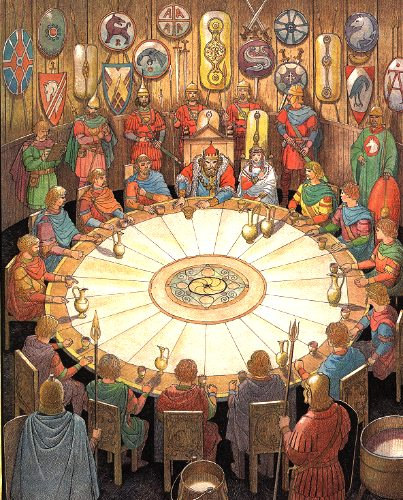Probability

# Discrete Probability: Level 4 ChallengesA man is stranded on an island. A benevolent genie presents three boxes, 23 white marbles, and 7 black marbles and instructs the man, "You may distribute the marbles into the boxes any way you see fit, but you must use all of the marbles. Once you finish, you will choose a box at random and then choose a marble from that box at random. If the marble is white, then I will help you escape from this place."

Assuming the man distributes the marbles in his best interest, what is the probability that he escapes the island?

$20$ men are held captive by a pirate lord, including two friends Jack and Tony.

The pirate decides to set $10$ of them free. The $20$ men are randomly divided into $10$ pairs. Each pair of men then flip a fair coin to decide who goes free.

The probability that both Jack and Tony are set free is $\frac{A}{B}$ where $A$ and $B$ are co-prime positive integers. Find the value of $A+B.$25 of King Arthur's knights are seated at the round table. Three of them are randomly chosen to be sent off to slay a troublesome dragon. Let $P$ be the probability that at least two of the three had been sitting next to each other. If $P$ can be expressed as $\frac {a}{b}$, where $a$ and $b$ are pairwise coprime integers, find $a+b$.

Suppose $8$ bugs are positioned at the $8$ corners of a unit cube, (one bug per corner). Each bug, simultaneously, randomly and independently, chooses one of the $3$ edges adjacent to its corner to travel on, and then does so until it reaches the next corner. (All the bugs travel at the same constant rate.)

The probability that none of the bugs meets any other bug in this process is $\dfrac{a}{b}$, where $a$ and $b$ are positive coprime integers. Find $a + b$.

A bag contains n marbles, some of which are red, the rest of which are white. If you were to draw two marbles (without replacement) from the bag, you'd be just as likely to get different-colored marbles as you would be to get marbles that were the same color. What is the largest possible value of n strictly less than 1000?

×